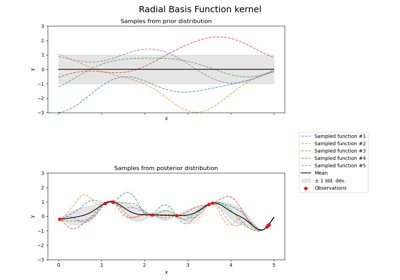# sklearn.gaussian_process.kernels.Matern¶

class sklearn.gaussian_process.kernels.Matern(length_scale=1.0, length_scale_bounds=(1e-05, 100000.0), nu=1.5)[source]

Matern kernel.

The class of Matern kernels is a generalization of the RBF. It has an additional parameter $$\nu$$ which controls the smoothness of the resulting function. The smaller $$\nu$$, the less smooth the approximated function is. As $$\nu\rightarrow\infty$$, the kernel becomes equivalent to the RBF kernel. When $$\nu = 1/2$$, the Matérn kernel becomes identical to the absolute exponential kernel. Important intermediate values are $$\nu=1.5$$ (once differentiable functions) and $$\nu=2.5$$ (twice differentiable functions).

The kernel is given by:

$k(x_i, x_j) = \frac{1}{\Gamma(\nu)2^{\nu-1}}\Bigg( \frac{\sqrt{2\nu}}{l} d(x_i , x_j ) \Bigg)^\nu K_\nu\Bigg( \frac{\sqrt{2\nu}}{l} d(x_i , x_j )\Bigg)$

where $$d(\cdot,\cdot)$$ is the Euclidean distance, $$K_{\nu}(\cdot)$$ is a modified Bessel function and $$\Gamma(\cdot)$$ is the gamma function. See , Chapter 4, Section 4.2, for details regarding the different variants of the Matern kernel.

Read more in the User Guide.

New in version 0.18.

Parameters:
length_scalefloat or ndarray of shape (n_features,), default=1.0

The length scale of the kernel. If a float, an isotropic kernel is used. If an array, an anisotropic kernel is used where each dimension of l defines the length-scale of the respective feature dimension.

length_scale_boundspair of floats >= 0 or “fixed”, default=(1e-5, 1e5)

The lower and upper bound on ‘length_scale’. If set to “fixed”, ‘length_scale’ cannot be changed during hyperparameter tuning.

nufloat, default=1.5

The parameter nu controlling the smoothness of the learned function. The smaller nu, the less smooth the approximated function is. For nu=inf, the kernel becomes equivalent to the RBF kernel and for nu=0.5 to the absolute exponential kernel. Important intermediate values are nu=1.5 (once differentiable functions) and nu=2.5 (twice differentiable functions). Note that values of nu not in [0.5, 1.5, 2.5, inf] incur a considerably higher computational cost (appr. 10 times higher) since they require to evaluate the modified Bessel function. Furthermore, in contrast to l, nu is kept fixed to its initial value and not optimized.

Attributes:
anisotropic
bounds

Returns the log-transformed bounds on the theta.

hyperparameter_length_scale
hyperparameters

Returns a list of all hyperparameter specifications.

n_dims

Returns the number of non-fixed hyperparameters of the kernel.

requires_vector_input

Returns whether the kernel is defined on fixed-length feature vectors or generic objects.

theta

Returns the (flattened, log-transformed) non-fixed hyperparameters.

References

Examples

>>> from sklearn.datasets import load_iris
>>> from sklearn.gaussian_process import GaussianProcessClassifier
>>> from sklearn.gaussian_process.kernels import Matern
>>> kernel = 1.0 * Matern(length_scale=1.0, nu=1.5)
>>> gpc = GaussianProcessClassifier(kernel=kernel,
...         random_state=0).fit(X, y)
>>> gpc.score(X, y)
0.9866...
>>> gpc.predict_proba(X[:2,:])
array([[0.8513..., 0.0368..., 0.1117...],
[0.8086..., 0.0693..., 0.1220...]])


Methods

 __call__(X[, Y, eval_gradient]) Return the kernel k(X, Y) and optionally its gradient. clone_with_theta(theta) Returns a clone of self with given hyperparameters theta. Returns the diagonal of the kernel k(X, X). get_params([deep]) Get parameters of this kernel. Returns whether the kernel is stationary. set_params(**params) Set the parameters of this kernel.

Return the kernel k(X, Y) and optionally its gradient.

Parameters:
Xndarray of shape (n_samples_X, n_features)

Left argument of the returned kernel k(X, Y)

Yndarray of shape (n_samples_Y, n_features), default=None

Right argument of the returned kernel k(X, Y). If None, k(X, X) if evaluated instead.

Determines whether the gradient with respect to the log of the kernel hyperparameter is computed. Only supported when Y is None.

Returns:
Kndarray of shape (n_samples_X, n_samples_Y)

Kernel k(X, Y)

K_gradientndarray of shape (n_samples_X, n_samples_X, n_dims), optional

The gradient of the kernel k(X, X) with respect to the log of the hyperparameter of the kernel. Only returned when eval_gradient is True.

property bounds

Returns the log-transformed bounds on the theta.

Returns:
boundsndarray of shape (n_dims, 2)

The log-transformed bounds on the kernel’s hyperparameters theta

clone_with_theta(theta)[source]

Returns a clone of self with given hyperparameters theta.

Parameters:
thetandarray of shape (n_dims,)

The hyperparameters

diag(X)[source]

Returns the diagonal of the kernel k(X, X).

The result of this method is identical to np.diag(self(X)); however, it can be evaluated more efficiently since only the diagonal is evaluated.

Parameters:
Xndarray of shape (n_samples_X, n_features)

Left argument of the returned kernel k(X, Y)

Returns:
K_diagndarray of shape (n_samples_X,)

Diagonal of kernel k(X, X)

get_params(deep=True)[source]

Get parameters of this kernel.

Parameters:
deepbool, default=True

If True, will return the parameters for this estimator and contained subobjects that are estimators.

Returns:
paramsdict

Parameter names mapped to their values.

property hyperparameters

Returns a list of all hyperparameter specifications.

is_stationary()[source]

Returns whether the kernel is stationary.

property n_dims

Returns the number of non-fixed hyperparameters of the kernel.

property requires_vector_input

Returns whether the kernel is defined on fixed-length feature vectors or generic objects. Defaults to True for backward compatibility.

set_params(**params)[source]

Set the parameters of this kernel.

The method works on simple kernels as well as on nested kernels. The latter have parameters of the form <component>__<parameter> so that it’s possible to update each component of a nested object.

Returns:
self
property theta

Returns the (flattened, log-transformed) non-fixed hyperparameters.

Note that theta are typically the log-transformed values of the kernel’s hyperparameters as this representation of the search space is more amenable for hyperparameter search, as hyperparameters like length-scales naturally live on a log-scale.

Returns:
thetandarray of shape (n_dims,)

The non-fixed, log-transformed hyperparameters of the kernel

## Examples using sklearn.gaussian_process.kernels.Matern¶Illustration of prior and posterior Gaussian process for different kernels

Illustration of prior and posterior Gaussian process for different kernels